## Describing Data

Once we have collected data from surveys or experiments, we need to summarize and present the data in a way that will be meaningful to the reader. We will begin with graphical presentations of data then explore numerical summaries of data.

## Presenting Categorical Data Graphically

Categorical, or qualitative, data are pieces of information that allow us to classify the objects under investigation into various categories. We usually begin working with categorical data by summarizing the data into a frequency table.

### Frequency Table

A frequency table is a table with two columns. One column lists the categories, and another for the frequencies with which the items in the categories occur (how many items fit into each category).

### Example 1

An insurance company determines vehicle insurance premiums based on known risk factors. If a person is considered a higher risk, their premiums will be higher. One potential factor is the color of your car. The insurance company believes that people with some color cars are more likely to get in accidents. To research this, they examine police reports for recent total-loss collisions. The data is summarized in the frequency table below.

Color Frequency
Blue 25
Green 52
Red 41
White 36
Black 39
Grey 23

Sometimes we need an even more intuitive way of displaying data. This is where charts and graphs come in. There are many, many ways of displaying data graphically, but we will concentrate on one very useful type of graph called a bar graph. In this section we will work with bar graphs that display categorical data; the next section will be devoted to bar graphs that display quantitative data.

### Bar Graph

A bar graph is a graph that displays a bar for each category with the length of each bar indicating the frequency of that category.

To construct a bar graph, we need to draw a vertical axis and a horizontal axis. The vertical direction will have a scale and measure the frequency of each category; the horizontal axis has no scale in this instance. The construction of a bar chart is most easily described by use of an example.

### Example 2

Using our car data from above, note the highest frequency is 52, so our vertical axis needs to go from 0 to 52, but we might as well use 0 to 55, so that we can put a hash mark every 5 units: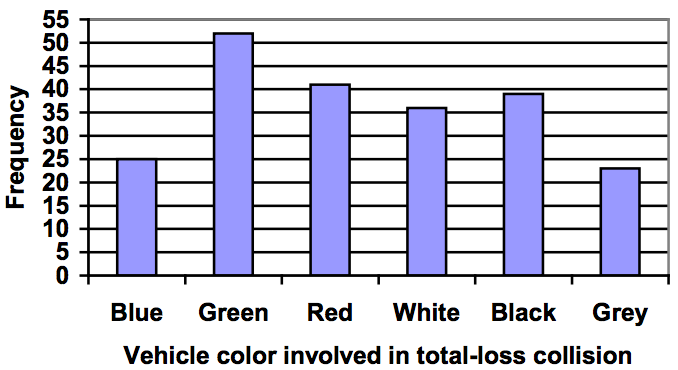Notice that the height of each bar is determined by the frequency of the corresponding color. The horizontal gridlines are a nice touch, but not necessary. In practice, you will find it useful to draw bar graphs using graph paper, so the gridlines will already be in place, or using technology. Instead of gridlines, we might also list the frequencies at the top of each bar, like this: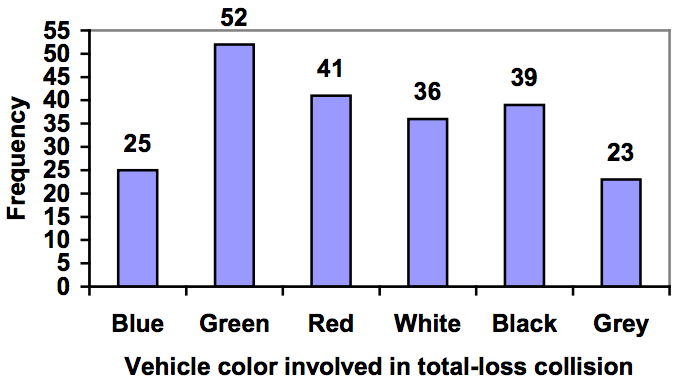In this case, our chart might benefit from being reordered from largest to smallest frequency values. This arrangement can make it easier to compare similar values in the chart, even without gridlines. When we arrange the categories in decreasing frequency order like this, it is called a Pareto chart.

### Pareto Chart

A Pareto chart is a bar graph ordered from highest to lowest frequency

### Example 3

Transforming our bar graph from earlier into a Pareto chart, we get: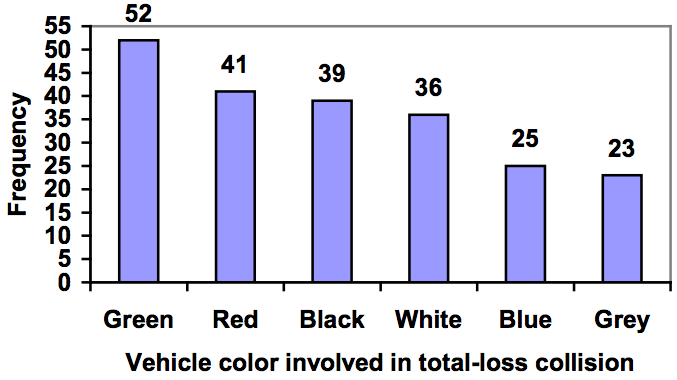### Example 4

In a survey, adults were asked whether they personally worried about a variety of environmental concerns. The numbers (out of 1012 surveyed) who indicated that they worried “a great deal” about some selected concerns are summarized below.

Environmental Issue Frequency
Pollution of drinking water 597
Contamination of soil and water by toxic waste 526
Air pollution 455
Global warming 354

This data could be shown graphically in a bar graph: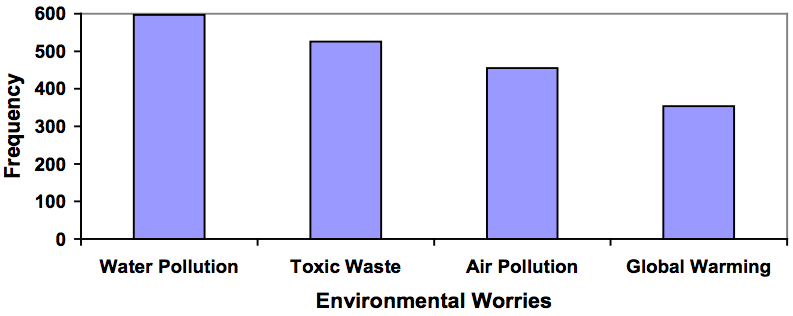To show relative sizes, it is common to use a pie chart.

### Pie Chart

A pie chart is a circle with wedges cut of varying sizes marked out like slices of pie or pizza. The relative sizes of the wedges correspond to the relative frequencies of the categories.

### Example 5

For our vehicle color data, a pie chart might look like this: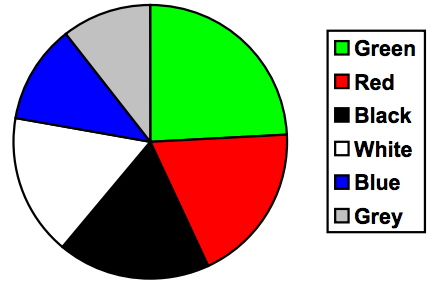Pie charts can often benefit from including frequencies or relative frequencies (percents) in the chart next to the pie slices. Often having the category names next to the pie slices also makes the chart clearer.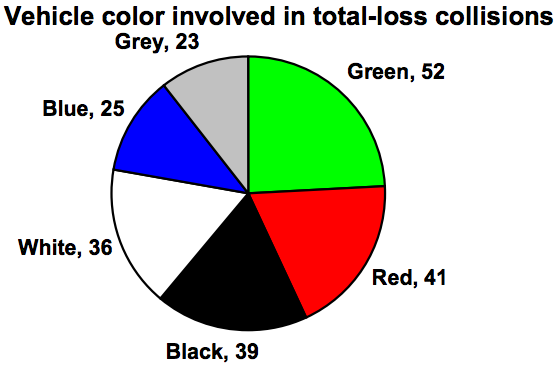### Example 6

The pie chart to the right shows the percentage of voters supporting each candidate running for a local senate seat.

If there are 20,000 voters in the district, the pie chart shows that about 11% of those, about 2,200 voters, support Reeves.

Pie charts look nice, but are harder to draw by hand than bar charts since to draw them accurately we would need to compute the angle each wedge cuts out of the circle, then measure the angle with a protractor. Computers are much better suited to drawing pie charts. Common software programs like Microsoft Word or Excel, OpenOffice.org Write or Calc, or Google Docs are able to create bar graphs, pie charts, and other graph types. There are also numerous online tools that can create graphs.

### Try It Now

Create a bar graph and a pie chart to illustrate the grades on a history exam below.

A: 12 students, B: 19 students, C: 14 students, D: 4 students, F: 5 students

Don’t get fancy with graphs! People sometimes add features to graphs that don’t help to convey their information. For example, 3-dimensional bar charts like the one shown below are usually not as effective as their two-dimensional counterparts.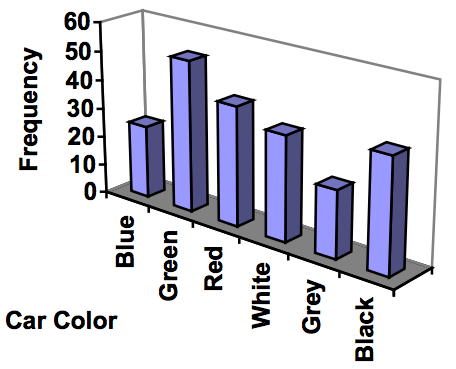Here is another way that fanciness can lead to trouble. Instead of plain bars, it is tempting to substitute meaningful images. This type of graph is called a pictogram.

### Pictogram

A pictogram is a statistical graphic in which the size of the picture is intended to represent the frequencies or size of the values being represented.

### Example 7A labor union might produce the graph to the right to show the difference between the average manager salary and the average worker salary.

Looking at the picture, it would be reasonable to guess that the manager salaries is 4 times as large as the worker salaries—the area of the bag looks about 4 times as large. However, the manager salaries are in fact only twice as large as worker salaries, which were reflected in the picture by making the manager bag twice as tall.

Another distortion in bar charts results from setting the baseline to a value other than zero. The baseline is the bottom of the vertical axis, representing the least number of cases that could have occurred in a category. Normally, this number should be zero.

### Example 8

Compare the two graphs below showing support for same-sex marriage rights from a poll taken in December 2008. The difference in the vertical scale on the first graph suggests a different story than the true differences in percentages; the second graph makes it look like twice as many people oppose marriage rights as support it.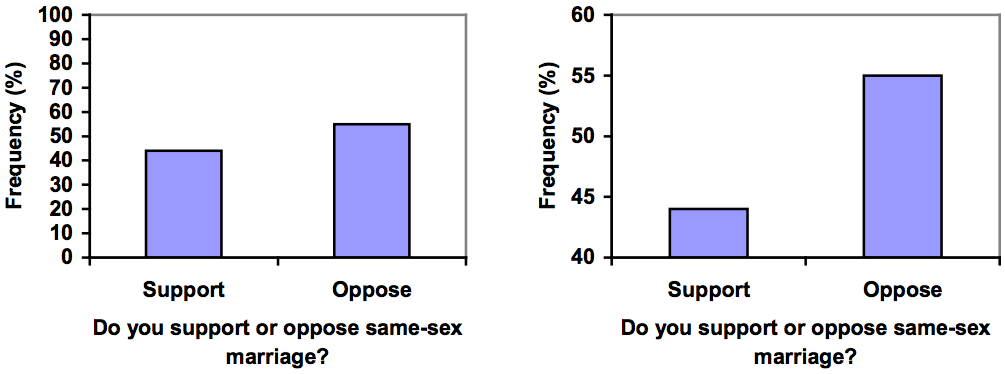### Try It Now

A poll was taken asking people if they agreed with the positions of the 4 candidates for a county office. Does the pie chart present a good representation of this data? Explain.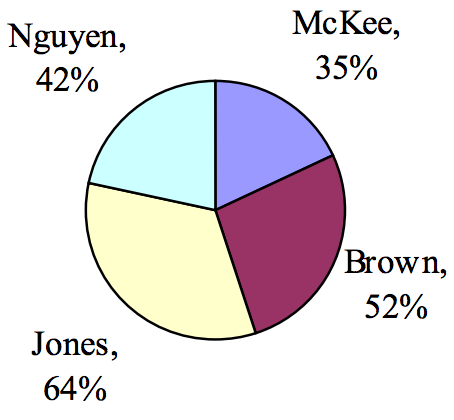## Presenting Quantitative Data Graphically

Quantitative, or numerical, data can also be summarized into frequency tables.

### Example 9

A teacher records scores on a 20-point quiz for the 30 students in his class. The scores are:

19 20 18 18 17 18 19 17 20 18 20 16 20 15 17 12 18 19 18 19 17 20 18 16 15 18 20 5 0 0

These scores could be summarized into a frequency table by grouping like values:

Score Frequency
0 2
5 1
12 1
15 2
16 2
17 4
18 8
19 4
20 6

Using this table, it would be possible to create a standard bar chart from this summary, like we did for categorical data: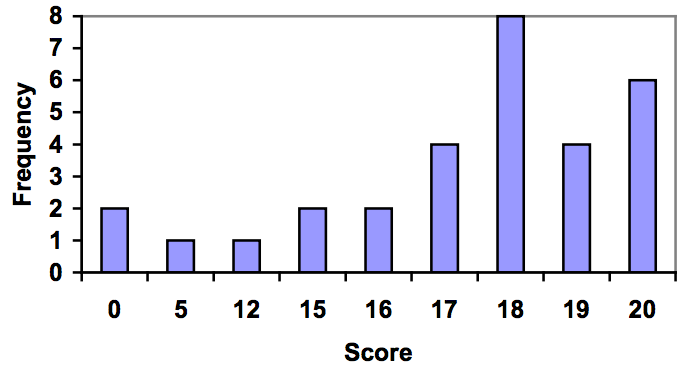However, since the scores are numerical values, this chart doesn’t really make sense; the first and second bars are five values apart, while the later bars are only one value apart. It would be more correct to treat the horizontal axis as a number line. This type of graph is called a histogram.

### Histogram

A histogram is like a bar graph, but where the horizontal axis is a number line

### Example 10

For the values above, a histogram would look like: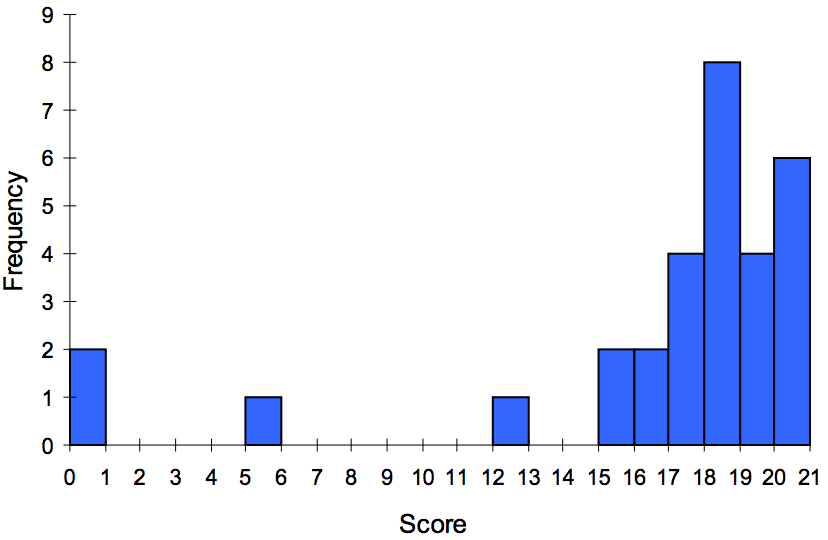Notice that in the histogram, a bar represents values on the horizontal axis from that on the left hand-side of the bar up to, but not including, the value on the right hand side of the bar. Some people choose to have bars start at ½ values to avoid this ambiguity.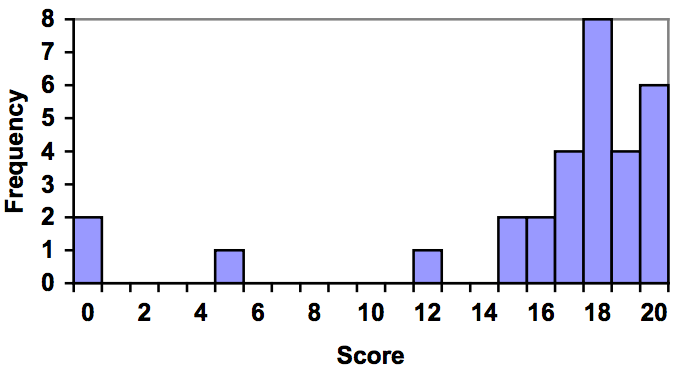Unfortunately, not a lot of common software packages can correctly graph a histogram. About the best you can do in Excel or Word is a bar graph with no gap between the bars and spacing added to simulate a numerical horizontal axis.

If we have a large number of widely varying data values, creating a frequency table that lists every possible value as a category would lead to an exceptionally long frequency table, and probably would not reveal any patterns. For this reason, it is common with quantitative data to group data into class intervals.

### Class Intervals

Class intervals are groupings of the data. In general, we define class intervals so that:

• Each interval is equal in size. For example, if the first class contains values from 120 to 129, the second class should include values from 130 to 139.
• We have somewhere between 5 and 20 classes, typically, depending upon the number of data we’re working with.

### Example 11

Suppose that we have collected weights from 100 male subjects as part of a nutrition study. For our weight data, we have values ranging from a low of 121 pounds to a high of 263 pounds, giving a total span of 263-121 = 142. We could create 7 intervals with a width of around 20, 14 intervals with a width of around 10, or somewhere in between. Often time we have to experiment with a few possibilities to find something that represents the data well. Let us try using an interval width of 15. We could start at 121, or at 120 since it is a nice round number.

Interval Frequency
120–134 4
135–149 14
150–164 16
165–179 28
180–194 12
195–209 8
210–224 7
225–239 6
240–254 2
255–269 3

A histogram of this data would look like: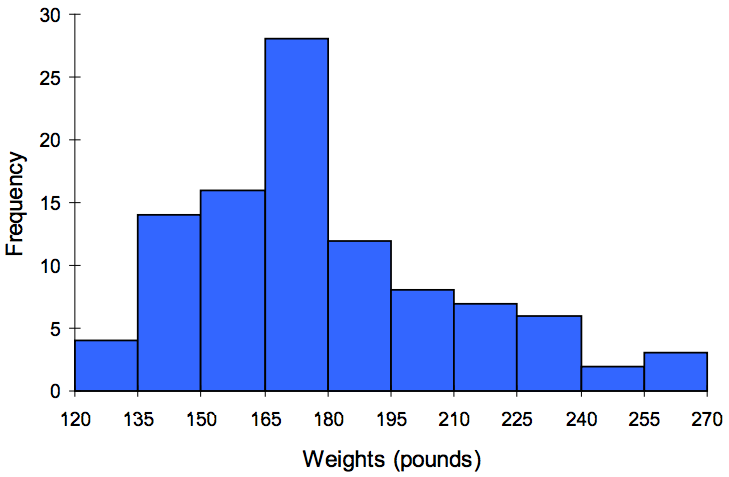In many software packages, you can create a graph similar to a histogram by putting the class intervals as the labels on a bar chart.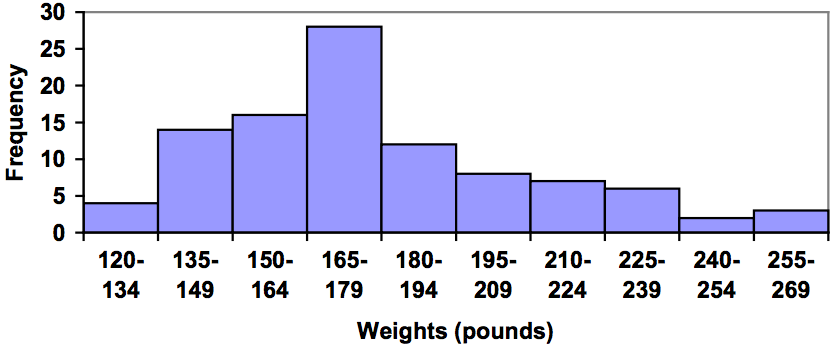Other graph types such as pie charts are possible for quantitative data. The usefulness of different graph types will vary depending upon the number of intervals and the type of data being represented. For example, a pie chart of our weight data is difficult to read because of the quantity of intervals we used.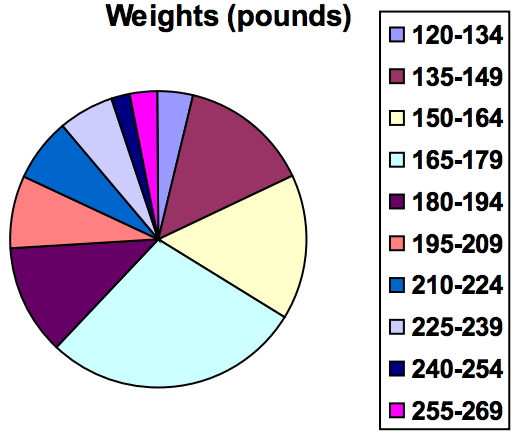### Try It Now

The total cost of textbooks for the term was collected from 36 students. Create a histogram for this data.

 $140$160 $160$165 $180$220 $235$240 $250$260 $280$285 $285$285 $290$300 $300$305 $310$310 $315$315 $320$320 $330$340 $345$350 $355$360 $360$380 $395$420 $460$460

When collecting data to compare two groups, it is desirable to create a graph that compares quantities.

### Example 12

The data below came from a task in which the goal is to move a computer mouse to a target on the screen as fast as possible. On 20 of the trials, the target was a small rectangle; on the other 20, the target was a large rectangle. Time to reach the target was recorded on each trial.

Interval
(milliseconds)
Frequency:
small target
Frequency:
large target
300–399 0 0
400–499 1 5
500–599 3 10
600–699 6 5
700–799 5 0
800–899 4 0
900–999 0 0
1000–1099 1 0
1100–1199 0 0

One option to represent this data would be a comparative histogram or bar chart, in which bars for the small target group and large target group are placed next to each other.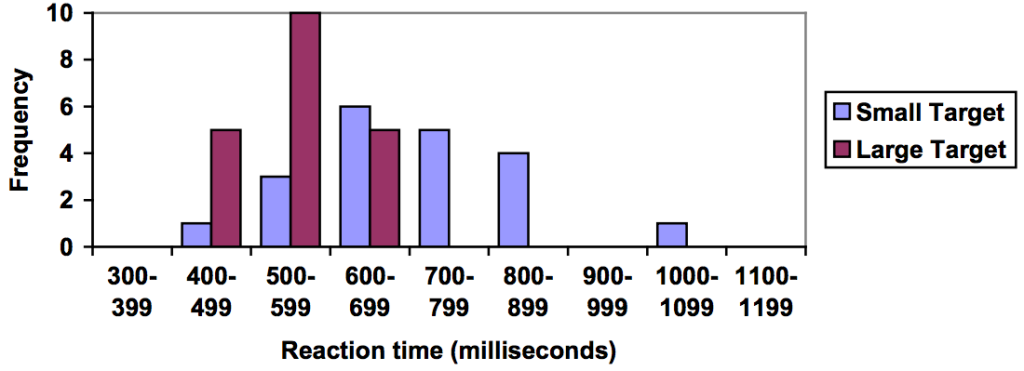### Frequency Polygon

An alternative representation is a frequency polygon. A frequency polygon starts out like a histogram, but instead of drawing a bar, a point is placed in the midpoint of each interval at height equal to the frequency. Typically the points are connected with straight lines to emphasize the distribution of the data.

### Example 13

This graph makes it easier to see that reaction times were generally shorter for the larger target, and that the reaction times for the smaller target were more spread out.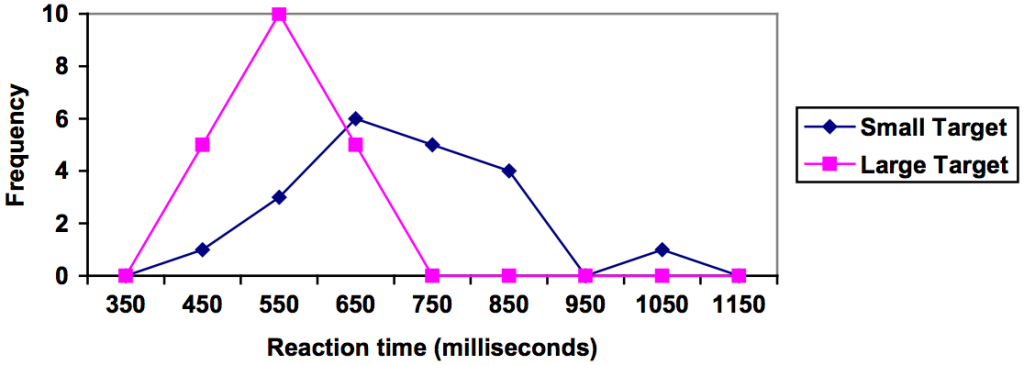## Numerical Summaries of Data

It is often desirable to use a few numbers to summarize a distribution. One important aspect of a distribution is where its center is located. Measures of central tendency are discussed first. A second aspect of a distribution is how spread out it is. In other words, how much the data in the distribution vary from one another. The second section describes measures of variability.

## Measures of Central Tendency

Let’s begin by trying to find the most “typical” value of a data set.

Note that we just used the word “typical” although in many cases you might think of using the word “average.” We need to be careful with the word “average” as it means different things to different people in different contexts. One of the most common uses of the word “average” is what mathematicians and statisticians call the arithmetic mean, or just plain old mean for short. “Arithmetic mean” sounds rather fancy, but you have likely calculated a mean many times without realizing it; the mean is what most people think of when they use the word “average”.

### Mean

The mean of a set of data is the sum of the data values divided by the number of values.

### Example 14

Marci’s exam scores for her last math class were: 79, 86, 82, 94. The mean of these values would be:

$\frac{79+86+82+94}{4}=85.25\\$. Typically we round means to one more decimal place than the original data had. In this case, we would round 85.25 to 85.3.

### Example 15

The number of touchdown (TD) passes thrown by each of the 31 teams in the National Football League in the 2000 season are shown below.

37 33 33 32 29 28 28 23 22 22 22 21 21 21 20
20 19 19 18 18 18 18 16 15 14 14 14 12 12 9 6

Adding these values, we get 634 total TDs. Dividing by 31, the number of data values, we get $\frac{634}{31}=20.4516\\$. It would be appropriate to round this to 20.5.

It would be most correct for us to report that “The mean number of touchdown passes thrown in the NFL in the 2000 season was 20.5 passes,” but it is not uncommon to see the more casual word “average” used in place of “mean.”

The price of a jar of peanut butter at 5 stores was: $3.29,$3.59, $3.79,$3.75, and $3.99. Find the mean price. ### Example 16 The one hundred families in a particular neighborhood are asked their annual household income, to the nearest$5 thousand dollars. The results are summarized in a frequency table below.

Income (thousands of dollars) Frequency
15 6
20 8
25 11
30 17
35 19
40 20
45 12
50 7

Calculating the mean by hand could get tricky if we try to type in all 100 values:

$\displaystyle\frac{\stackrel{6\text{ terms}}{\overbrace{15+\cdots{+}15}}+\stackrel{8\text{ terms}}{\overbrace{20+\cdots{+}20}}+\stackrel{11\text{ terms}}{\overbrace{25+\cdots{+}25}}+\cdots}{100}$

We could calculate this more easily by noticing that adding 15 to itself six times is the same as 15 × 6 = 90. Using this simplification, we get

$\frac{15\cdot6+20\cdot8+25\cdot11+30\cdot17+35\cdot19+40\cdot20+\cdot12+50\cdot7}{100}=\frac{3390}{100}=33.9\\$

The mean household income of our sample is 33.9 thousand dollars ($33,900). ### Example 17 Extending off the last example, suppose a new family moves into the neighborhood example that has a household income of$5 million ($5000 thousand). Adding this to our sample, our mean is now: $\frac{15\cdot6+20\cdot8+25\cdot11+30\cdot17+35\cdot19+40\cdot20+\cdot12+50\cdot7+5000\cdot1}{101}=\frac{3390}{101}=83.069\\$ While 83.1 thousand dollars ($83,069) is the correct mean household income, it no longer represents a “typical” value.

Imagine the data values on a see-saw or balance scale. The mean is the value that keeps the data in balance, like in the picture below.### Example 20

Let us return now to our original household income data:

Income (thousands of dollars) Frequency
15 6
20 8
25 11
30 17
35 19
40 20
45 12
50 7

Here we have 100 data values. If we didn’t already know that, we could find it by adding the frequencies. Since 100 is an even number, we need to find the mean of the middle two data values: the 50th and 51st data values. To find these, we start counting up from the bottom:

There are 6 data values of $15, so values 1 to 6 are$15 thousand
The next 8 data values are $20, so values 7 to (6+8)=14 are$20 thousand
The next 11 data values are $25, so values 15 to (14+11)=25 are$25 thousand
The next 17 data values are $30, so values 26 to (25+17)=42 are$30 thousand
The next 19 data values are $35, so values 43 to (42+19)=61 are$35 thousand

From this we can tell that values 50 and 51 will be $35 thousand, and the mean of these two values is$35 thousand. The median income in this neighborhood is $35 thousand. ### Example 21 If we add in the new neighbor with a$5 million household income, then there will be 101 data values, and the 51st value will be the median. As we discovered in the last example, the 51st value is $35 thousand. Notice that the new neighbor did not affect the median in this case. The median is not swayed as much by outliers as the mean is. In addition to the mean and the median, there is one other common measurement of the “typical” value of a data set: the mode. ### Mode The mode is the element of the data set that occurs most frequently. The mode is fairly useless with data like weights or heights where there are a large number of possible values. The mode is most commonly used for categorical data, for which median and mean cannot be computed. ### Example 22 In our vehicle color survey, we collected the data Color Frequency Blue 3 Green 5 Red 4 White 3 Black 2 Grey 3 For this data, Green is the mode, since it is the data value that occurred the most frequently. It is possible for a data set to have more than one mode if several categories have the same frequency, or no modes if each every category occurs only once. ### Try It Now Reviewers were asked to rate a product on a scale of 1 to 5. Find 1. The mean rating 2. The median rating 3. The mode rating Rating Frequency 1 4 2 8 3 7 4 3 5 1 ## Measures of Variation Consider these three sets of quiz scores: Section A: 5 5 5 5 5 5 5 5 5 5 Section B: 0 0 0 0 0 10 10 10 10 10 Section C: 4 4 4 5 5 5 5 6 6 6 All three of these sets of data have a mean of 5 and median of 5, yet the sets of scores are clearly quite different. In section A, everyone had the same score; in section B half the class got no points and the other half got a perfect score, assuming this was a 10-point quiz. Section C was not as consistent as section A, but not as widely varied as section B. In addition to the mean and median, which are measures of the “typical” or “middle” value, we also need a measure of how “spread out” or varied each data set is. There are several ways to measure this “spread” of the data. The first is the simplest and is called the range. ### Range The range is the difference between the maximum value and the minimum value of the data set. ### Example 23 Using the quiz scores from above, • For section A, the range is 0 since both maximum and minimum are 5 and 5 – 5 = 0 • For section B, the range is 10 since 10 – 0 = 10 • For section C, the range is 2 since 6 – 4 = 2 In the last example, the range seems to be revealing how spread out the data is. However, suppose we add a fourth section, Section D, with scores 0 5 5 5 5 5 5 5 5 10. This section also has a mean and median of 5. The range is 10, yet this data set is quite different than Section B. To better illuminate the differences, we’ll have to turn to more sophisticated measures of variation. ### Standard Deviation The standard deviation is a measure of variation based on measuring how far each data value deviates, or is different, from the mean. A few important characteristics: • Standard deviation is always positive. Standard deviation will be zero if all the data values are equal, and will get larger as the data spreads out. • Standard deviation has the same units as the original data. • Standard deviation, like the mean, can be highly influenced by outliers. Using the data from section D, we could compute for each data value the difference between the data value and the mean: data value deviation: data value − mean 0 0 − 5 = −5 5 5 − 5 = 0 5 5 − 5 = 0 5 5 − 5 = 0 5 5 − 5 = 0 5 5 − 5 = 0 5 5 − 5 = 0 5 5 − 5 = 0 5 5 − 5 = 0 10 10 − 5 = 5 We would like to get an idea of the “average” deviation from the mean, but if we find the average of the values in the second column the negative and positive values cancel each other out (this will always happen), so to prevent this we square every value in the second column: data value deviation: data value − mean deviation squared 0 0−5 = −5 (−5)2 = 25 5 5 − 5 = 0 02 = 0 5 5-5 = 0 02 = 0 5 5 − 5 = 0 02 = 0 5 5 − 5 = 0 02 = 0 5 5 − 5 = 0 02 = 0 5 5 − 5 = 0 02 = 0 5 5 − 5 = 0 02 = 0 5 5 − 5 = 0 02 = 0 10 10 − 5 = 5 (5)2 = 25 We then add the squared deviations up to get 25 + 0 + 0 + 0 + 0 + 0 + 0 + 0 + 0 + 25 = 50. Ordinarily we would then divide by the number of scores, n, (in this case, 10) to find the mean of the deviations. But we only do this if the data set represents a population; if the data set represents a sample (as it almost always does), we instead divide by n − 1 (in this case, 10 − 1 = 9). So in our example, we would have 50/10 = 5 if section D represents a population and 50/9 = about 5.56 if section D represents a sample. These values (5 and 5.56) are called, respectively, the population variance and the sample variance for section D. Variance can be a useful statistical concept, but note that the units of variance in this instance would be points-squared since we squared all of the deviations. What are points-squared? Good question. We would rather deal with the units we started with (points in this case), so to convert back we take the square root and get: population standard deviation $=\sqrt{\frac{50}{10}}=\sqrt{5}\approx{2.2}\\$ or population standard deviation $=\sqrt{\frac{50}{9}}\approx{2.4}\\$ If we are unsure whether the data set is a sample or a population, we will usually assume it is a sample, and we will round answers to one more decimal place than the original data, as we have done above. ### To compute standard deviation: 1. Find the deviation of each data from the mean. In other words, subtract the mean from the data value. 2. Square each deviation. 3. Add the squared deviations. 4. Divide by n, the number of data values, if the data represents a whole population; divide by n − 1 if the data is from a sample. 5. Compute the square root of the result. ### Example 24 Computing the standard deviation for Section B above, we first calculate that the mean is 5. Using a table can help keep track of your computations for the standard deviation: data value deviation: data value − mean deviation squared 0 0 − 5 = −5 (−5)2 = 25 0 0 − 5 = −5 (−5)2 = 25 0 0 − 5 = −5 (−5)2 = 25 0 0 − 5 = −5 (−5)2 = 25 0 0 − 5 = −5 (−5)2 = 25 10 10 − 5 = −5 (5)2 = 25 10 10 − 5 = −5 (5)2 = 25 10 10 − 5 = −5 (5)2 = 25 10 10 − 5 = −5 (5)2 = 25 10 10 − 5 = −5 (5)2 = 25 Assuming this data represents a population, we will add the squared deviations, divide by 10, the number of data values, and compute the square root: $\displaystyle\sqrt{\frac{25+25+25+25+25+25+25+25+25+25}{10}}=\sqrt{\frac{250}{10}}=5\\$ Notice that the standard deviation of this data set is much larger than that of section D since the data in this set is more spread out. For comparison, the standard deviations of all four sections are:  Section A: 5 5 5 5 5 5 5 5 5 5 Standard deviation: 0 Section B: 0 0 0 0 0 10 10 10 10 10 Standard deviation: 5 Section C: 4 4 4 5 5 5 5 6 6 6 Standard deviation: 0.8 Section D: 0 5 5 5 5 5 5 5 5 10 Standard deviation: 2.2 ### Try It Now The price of a jar of peanut butter at 5 stores were:$3.29, $3.59,$3.79, $3.75, and$3.99. Find the standard deviation of the prices.

Where standard deviation is a measure of variation based on the mean, quartiles are based on the median.

### Quartiles

Quartiles are values that divide the data in quarters.

The first quartile (Q1) is the value so that 25% of the data values are below it; the third quartile (Q3) is the value so that 75% of the data values are below it. You may have guessed that the second quartile is the same as the median, since the median is the value so that 50% of the data values are below it.

This divides the data into quarters; 25% of the data is between the minimum and Q1, 25% is between Q1 and the median, 25% is between the median and Q3, and 25% is between Q3 and the maximum value

While quartiles are not a 1-number summary of variation like standard deviation, the quartiles are used with the median, minimum, and maximum values to form a 5 number summary of the data.

### Five number summary

The five number summary takes this form:

Minimum, Q1, Median, Q3, Maximum

To find the first quartile, we need to find the data value so that 25% of the data is below it. If n is the number of data values, we compute a locator by finding 25% of n. If this locator is a decimal value, we round up, and find the data value in that position. If the locator is a whole number, we find the mean of the data value in that position and the next data value. This is identical to the process we used to find the median, except we use 25% of the data values rather than half the data values as the locator.

### To find the first quartile, Q1

Begin by ordering the data from smallest to largest

Compute the locator: L = 0.25n

If L is a decimal value:

• Round up to L+
• Use the data value in the L+th position

If L is a whole number:

• Find the mean of the data values in the Lth and L + 1th positions.

### To find the third quartile, Q3

Use the same procedure as for Q1L = 0.75n

Examples should help make this clearer.

### Example 25

Suppose we have measured 9 females and their heights (in inches), sorted from smallest to largest are:

59 60 62 64 66 67 69 70 72

To find the first quartile we first compute the locator: 25% of 9 is L = 0.25(9) = 2.25. Since this value is not a whole number, we round up to 3. The first quartile will be the third data value: 62 inches.

To find the third quartile, we again compute the locator: 75% of 9 is 0.75(9) = 6.75. Since this value is not a whole number, we round up to 7. The third quartile will be the seventh data value: 69 inches.

### Example 26

Suppose we had measured 8 females and their heights (in inches), sorted from smallest to largest are:

59 60 62 64 66 67 69 70

To find the first quartile we first compute the locator: 25% of 8 is L = 0.25(8) = 2. Since this value is a whole number, we will find the mean of the 2nd and 3rd data values: (60+62)/2 = 61, so the first quartile is 61 inches.

The third quartile is computed similarly, using 75% instead of 25%. L = 0.75(8) = 6. This is a whole number, so we will find the mean of the 6th and 7th data values: (67+69)/2 = 68, so Q3 is 68.

Note that the median could be computed the same way, using 50%.

The 5-number summary combines the first and third quartile with the minimum, median, and maximum values.

### Example 27

For the 9 female sample, the median is 66, the minimum is 59, and the maximum is 72. The 5 number summary is: 59, 62, 66, 69, 72.

For the 8 female sample, the median is 65, the minimum is 59, and the maximum is 70, so the 5 number summary would be: 59, 61, 65, 68, 70.

### Example 28

Returning to our quiz score data. In each case, the first quartile locator is 0.25(10) = 2.5, so the first quartile will be the 3rd data value, and the third quartile will be the 8th data value. Creating the five-number summaries:

Section and data 5-number summary
Section A: 5 5 5 5 5 5 5 5 5 5 5, 5, 5, 5, 5
Section B: 0 0 0 0 0 10 10 10 10 10 0, 0, 5, 10, 10
Section C: 4 4 4 5 5 5 5 6 6 6 4, 4, 5, 6, 6
Section D: 0 5 5 5 5 5 5 5 5 10 0, 5, 5, 5, 10

Of course, with a relatively small data set, finding a five-number summary is a bit silly, since the summary contains almost as many values as the original data.

### Try It Now

The total cost of textbooks for the term was collected from 36 students. Find the 5 number summary of this data.

 $140$160 $160$165 $180$220 $235$240 $250$260 $280$285 $285$285 $290$300 $300$305 $310$310 $315$315 $320$320 $330$340 $345$350 $355$360 $360$380 $395$420 $460$460

### Example 29

Returning to the household income data from earlier, create the five-number summary.

Income (thousands of dollars) Frequency
15 6
20 8
25 11
30 17
35 19
40 20
45 12
50 7

By adding the frequencies, we can see there are 100 data values represented in the table. In Example 20, we found the median was $35 thousand. We can see in the table that the minimum income is$15 thousand, and the maximum is $50 thousand. To find Q1, we calculate the locator: L = 0.25(100) = 25. This is a whole number, so Q1 will be the mean of the 25th and 26th data values. Counting up in the data as we did before, There are 6 data values of$15, so values 1 to 6 are $15 thousand The next 8 data values are$20, so values 7 to (6+8)=14 are $20 thousand The next 11 data values are$25, so values 15 to (14+11)=25 are $25 thousand The next 17 data values are$30, so values 26 to (25+17)=42 are $30 thousand The 25th data value is$25 thousand, and the 26th data value is $30 thousand, so Q1 will be the mean of these: (25 + 30)/2 =$27.5 thousand.

To find Q3, we calculate the locator: L = 0.75(100) = 75. This is a whole number, so Q3 will be the mean of the 75th and 76th data values. Continuing our counting from earlier,

The next 19 data values are $35, so values 43 to (42+19)=61 are$35 thousand

The next 20 data values are $40, so values 61 to (61+20)=81 are$40 thousand

Both the 75th and 76th data values lie in this group, so Q3 will be $40 thousand. Putting these values together into a five-number summary, we get: 15, 27.5, 35, 40, 50 Note that the 5 number summary divides the data into four intervals, each of which will contain about 25% of the data. In the previous example, that means about 25% of households have income between$40 thousand and \$50 thousand.

For visualizing data, there is a graphical representation of a 5-number summary called a box plot, or box and whisker graph.

### Box Plot

A box plot is a graphical representation of a five-number summary.

To create a box plot, a number line is first drawn. A box is drawn from the first quartile to the third quartile, and a line is drawn through the box at the median. “Whiskers” are extended out to the minimum and maximum values.

### Example 30

The box plot below is based on the 9 female height data with 5 number summary: 59, 62, 66, 69, 72.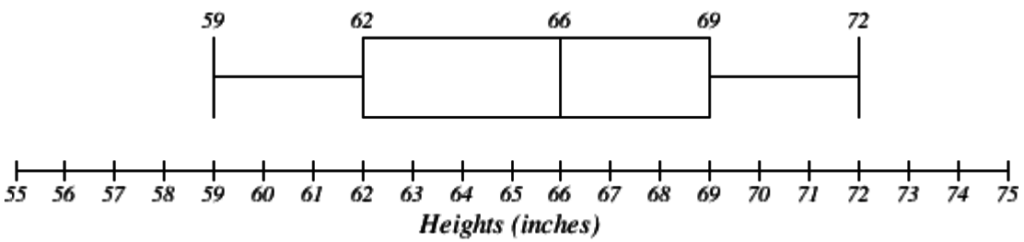### Example 31

The box plot below is based on the household income data with 5 number summary: 15, 27.5, 35, 40, 50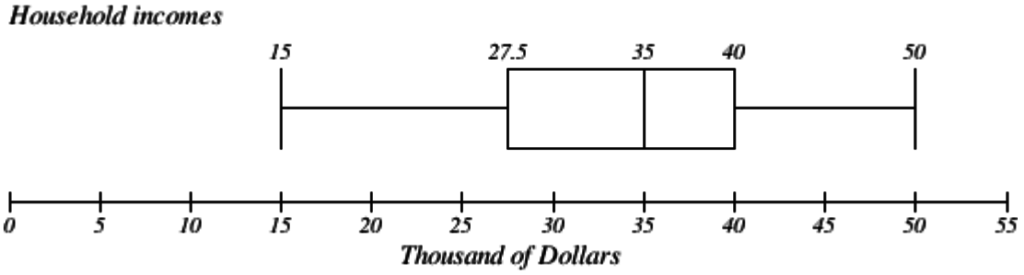### Try It Now

Create a boxplot based on the textbook price data from the last Try it Now.

Box plots are particularly useful for comparing data from two populations.

### Example 32

The box plot of service times for two fast-food restaurants is shown below.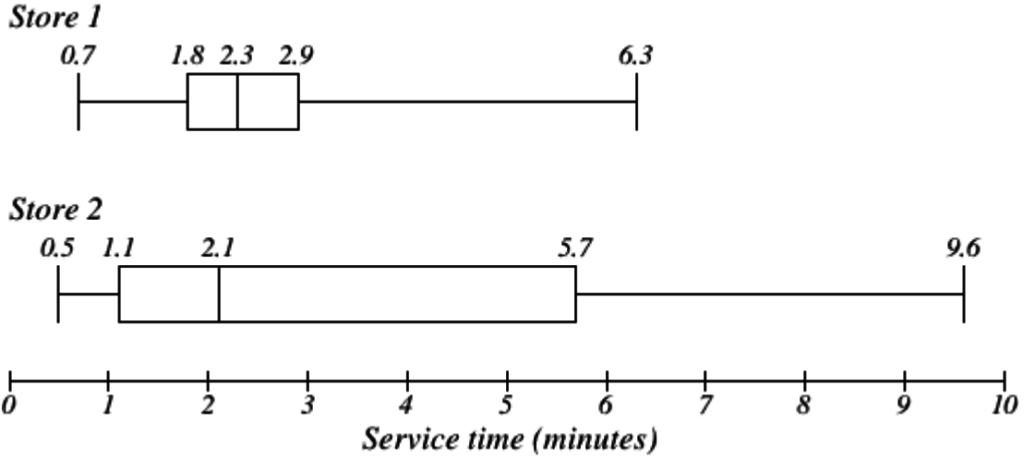While store 2 had a slightly shorter median service time (2.1 minutes vs. 2.3 minutes), store 2 is less consistent, with a wider spread of the data.

At store 1, 75% of customers were served within 2.9 minutes, while at store 2, 75% of customers were served within 5.7 minutes.

Which store should you go to in a hurry? That depends upon your opinions about luck: 25% of customers at store 2 had to wait between 5.7 and 9.6 minutes.

### Example 33

The boxplot below is based on the birth weights of infants with severe idiopathic respiratory distress syndrome (SIRDS). The boxplot is separated to show the birth weights of infants who survived and those that did not.

Comparing the two groups, the boxplot reveals that the birth weights of the infants that died appear to be, overall, smaller than the weights of infants that survived. In fact, we can see that the median birth weight of infants that survived is the same as the third quartile of the infants that died.

Similarly, we can see that the first quartile of the survivors is larger than the median weight of those that died, meaning that over 75% of the survivors had a birth weight larger than the median birth weight of those that died.

Looking at the maximum value for those that died and the third quartile of the survivors, we can see that over 25% of the survivors had birth weights higher than the heaviest infant that died.

The box plot gives us a quick, albeit informal, way to determine that birth weight is quite likely linked to survival of infants with SIRDS.

1. Gallup Poll. March 5–8, 2009. http://www.pollingreport.com/enviro.htm
2. For example: http://nces.ed.gov/nceskids/createAgraph/ or http://docs.google.com
3. CNN/Opinion Research Corporation Poll. Dec 19-21, 2008, from http://www.pollingreport.com/civil.htm
4. The reason we do this is highly technical, but we can see how it might be useful by considering the case of a small sample from a population that contains an outlier, which would increase the average deviation: the outlier very likely won't be included in the sample, so the mean deviation of the sample would underestimate the mean deviation of the population; thus we divide by a slightly smaller number to get a slightly bigger average deviation.
5. van Vliet, P.K. and Gupta, J.M. (1973) Sodium bicarbonate in idiopathic respiratory distress syndrome. Arch. Disease in Childhood, 48, 249–255. As quoted on http://openlearn.open.ac.uk/mod/oucontent/view.php?id=398296&section=1.1.3